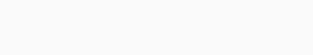# NewsCap Rates – a useful formula

With short term interest rates at multidecade highs and long term rates drifting up, and a lack of comparable transactions in the commercial property market, one wonders what capitalization rates should be going forwards. This post attempts to break down the capitalization rate theoretically based on a formula I encountered many years ago which I think may be useful today.

### A useful formula:Global Property Investment: Strategies, Structures, Decisions, First Edition. Andrew Baum, David Hartzell.© 2012 Andrew Baum and David Hartzell. Published 2012 by Blackwell Publishing Ltd. p 146

Where:
K: Cap Rate
RFR(r): Risk Free Rate, real
i: inflation
G(r): real growth of rents
d: depreciation

It basically says Cap rates = risk free rate (nominal) + risk premium – nominal rent growth + depreciation

Which raises some obvious questions:

1. What should the risk premium be? This probably depends on how you look at risk, whether you add up a bunch of risk factors (liquidity, location, property), look at historical risk premiums, or use some variation of finance CAPM based on volatility and return correlation. I certainly don’t know for any specific asset but in general I’d wager a guess most are around 1-5% (between AA investment grade bonds and equity market risk premiums).
2. What is a reasonable amount for depreciation? The book where I found the formula says probably 0.7-1.5%
3. But potentially more importantly now, what is long term nominal rent growth. Is there any real rent growth? And can real rent growth be negative – meaning nominal rent growth less than the inflation rate?

For this it’s interesting to think about what real rent growth means, for simplicity we’ll consider residential property. For rents to grow faster than inflation, it would mean at least one of these are happening:

1. That rents are increasing as a % of people’s incomes:
• This could happen as people get more wealthy and the basic necessities cost less, they can have more money to spend on housing. In essence, that foods and goods inflation < income growth < rent growth
• Preferences can also change, leading people to spend less on other things and more on housing
2. That incomes are growing through increased productivity growth:
• A more productive economy – nationally and locally – can lead to increased real incomes, and increased demand for housing

However, this only looks at housing demand. On the flip side is the supply of housing in a particular area. Although overabundance of housing supply can increase vacancy rates and decrease rental rates in the short run, there can be long term benefits to return returns from increased housing supply if you take into account agglomeration economics and the increased productivity and local incomes that can occur when a city or region expands and competes better on the global stage.

But different regions can have vastly different growth stories. And this has historically contributed to the differing cap rates between ‘core’ metropolitan areas and other places more out of the way. Now with Covid and work from home casting a shadow over the primacy downtown office cores. Needless to say there’s probably more than ever debate on how to think about risk premiums and real growth rates.

### Relation of Cap Rates to a DCF discount rate:

On a somewhat related note, given that this post is about Cap Rates, I’ve always thought cap rates and DCFs were similar given the similarity of the cap rate to the terminal value calculation of a DCF. And with some wolfram alpha I connect the two. For those interested in valuation math, read on.

Formula for a DCF:

PV = ∑ [ CFt / (1 + IRR)^t ]

Let’s pretend we have an unleveraged property and there’s no taxes, therefor:

NOI = CF

PV = NOI / (1 + IRR) + NOI / (1 + IRR)^2 + NOI / (1 + IRR)^3 + …

The formula for a the sum of an infinite geometric series is: a / (1-r)

Where:
a: the first term of the series
r: the ratio of two consecutive terms

a = noi/(1+irr)
r = noi/(1+irr)^2 / noi (1 + irr)
simplifying, r = 1/ (1+ irr)

Using the formula and wolfram alpha where n = noi and r = irr

Therefor, PV = NOI / IRR which looks like the cap rate formula, Value = NOI / Cap Rate.

The downside of cap rates is that NOI is constant, so if you’re expecting major changes in cash flows in the next 5-10 years then it may not be very useful to simply take an average long term rate which can be an oversimplification.

But it’s interesting that DCF models probably can be summarized as PV = CF / IRR.